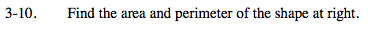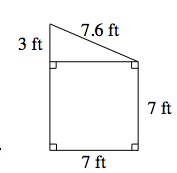### Home > MC2 > Chapter 3 > Lesson 3.1.1 > Problem3-10

3-10.Examine the separate parts of the shape individually as a triangle and a square. How can you use the measurements of these separate pieces to find the total area and perimeter of the overall shape?

Add up the areas of the triangle and the square to get the total area of the shape. Remember, area is base × height, but triangles have a special area calculation:

$\frac{1}{2} \text{(base }\times \text{ height).}$

Perimeter is the total distance around the boundary of a shape.

Area: 59.5 ft2
Solve for the perimeter by adding the side lengths.